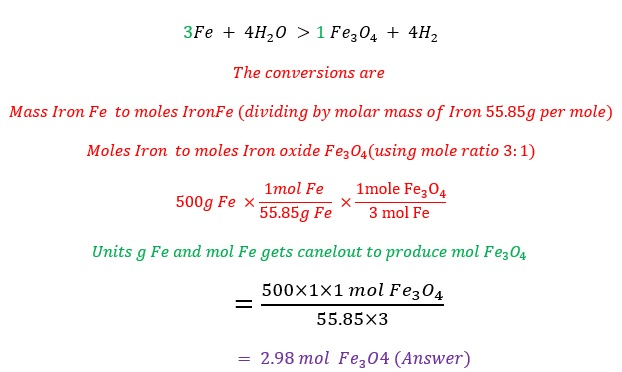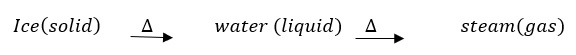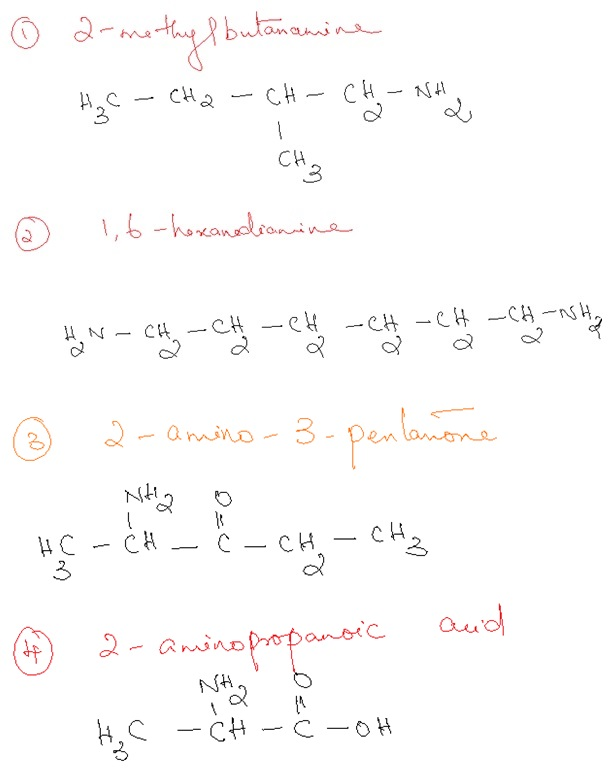# Chemistry Sample Assignment

1. For the reaction 3Fe + 4H2O ⟶ Fe3O4 + 4H2, how many moles of iron oxide are produced from 500 g of iron?

Solution : Balancing is making the number of atoms of each element same on both the sides (reactant and product side).

To find the number of atoms of each element we multiply coefficient * subscript

For example contains

5 ,Ca atoms and 5*2=10, Cl atoms

If there is a bracket in the chemical formula

For example we multiply coefficient * subscript * number outside the bracket.......... to find the number of atoms (Please note: 3 is the coefficient, and if there is no number given then 1 will be the coefficient )

So 3*3 =9 , Ca atoms

3*1*2=6, P atoms

3*4*2= 24, O atoms are present.

So

The balanced chemical equation is1. Two physical change in which the identitiy of a substance stays the same

Solution :Physical change is a change in which we see no change in the composition of the substance.

For example

On heating solid Ice, changes into liquid water. On further heating liquid water changes into water vapour(steam).Ice, water, steam all have the chemical formula H2O.

There is no change in the chemicla formula of the substance and no new substance gets formed.

A chemical change is the change in which a new substance gets formed. For example

3Fe + 4H20 > 1 Fe3 O4 + 4H2

here you see

Cutting and mixing are the two physical changes in which we see the identity of the substance remains same.(Answer)

Identity in the sense no change in the appearance or color of the material.

1. 3. The reaction between H2SO4 and KOH will generate a
soluble salt and water. True
or false?

An acid and a base react to produce salt and water which we call it as neutralization reaction.

The balanced chemical equation is

Here we see

Salts of Group I A elements are soluble in water. For example

We need to follow the solubility rules to find whether the salt is soluble or not.

Salts like acetates, nitrates, Group IA elements, ammonium salts are in general soluble in water.

Chlorides are soluble except chlorides of silver, lead, mercury.

Sulphides , phosphates , carbonates, hydroxides are generally insoluble salts with some exceptions.

1. __Be(OH)2=>__BeO+__H2O

Solution :

Balancing is making the number of atoms of each element same on both the sides (reactant and product side).

To find the number of atoms of each element we multiply coefficient * subscript

For example contains

5 ,Mg atoms and 5*2=10, Cl atoms

If there is a bracket in the chemical formula

For example we multiply coefficient * subscript * number outside the bracket.......... to find the number of atoms (Please note: 3 is the coefficient, and if there is no number given then 1 will be the coefficient )

So 3*3 =9 , Mg atoms

3*1*2=6, P atoms

3*4*2= 24, O atoms are present.

So

The balanced chemical equation is

 Reactant side (left) Elements present Product side (right) 1 2 2 Be O H 1 2 2 Balanced!!!!

Since the number of atoms of each element is same on both the sides the equation is already a balanced equation.

1. What are complex compounds ?

Irrelevant

1. What are the 2 atomic particles given off as a result of nuclear fission are _____ and _____. alpha beta gamma omega

Solution : alpha and beta particles .

Gamma is a ray. There is no particle like omega.

An alpha particles is identical to a Helium nucleus containing 2 protons and 2 neutrons.

A beta particle is identical to an electron. Beta decay,which the emission of an electron or a positron (a particle identical to an electron except that it has a positive electrical charge).

Many heavy nuclei emit an energetic alpha particle when they decay. For instance uranium-238 decays into thorium-234 with a half-life of almost 4.5 billion years by emitting an alpha particle.

Thorium-234,which is the decay product of uranium-238, in turn beta-decays into protactinium-234 by emitting an electron.

Heavy atoms such as uranium or plutonium can be split by bombarding them with neutrons. The resultant fragments, called fission products, are of intermediate atomic weight, and have a combined mass that is slightly smaller than the original nucleus. The difference appears as energy.

1. A balloon inflated in a room at 293 K has a volume of 4.0 L. The balloon then increases to 5.0 L. What is the new temperature if the pressure remains constant?

Solution :

According to Charle’s law,

For a given amount of gas at constant Pressure (P)

Volume is directly proportional to the temperature of the gas.

It is given by the expression

V α T ( at constant V and n)

V/T=k (k is a constant )

When there is a change in the volume or temperature

The expression is V1/T1 =V2/T2

The expression is

Where V1 and V2 are initial Volume and final Volume respectively
T1 and T2 are initial and final temperatures respectivley

Please Note: Directly proportional means when one increases the other one too will increase that is when volume increases temperature too will increases if Pressure is constant.

V1=4.0L
T1=293K
V2=5.0L
T2=?
rearranging the formula
T2=(V2 T1)/V1
Plugging in the values T2=(5.0L×293K)/4.0L=366.25K=366K or 93℃ (Answer)

Here we see as volume increases from 4.0 L to 5.0L temperature too increases from 293K to 366K.

1. 8. What is A for silicon tetrachloride sicl4

Irrelevant

1. The formula of a compound is M(OH)2. Given that the Mr of the compound is 98, calculate the Ar of M.
A)64
B)16
C)8
D)32

Solution :

Mr stands for the molecular mass of the compound which is =98

Ar stands for the atomic mass of the atom = ? we are asked to find.

Molecular mass of the compound =sum of the atomic mass of the atoms present in the molecular formula.

For example

```{`
CO2 is the molecular formula of Carbon dioxide
Molecular mass calculations:
1,C atom =12.01 amu
2,O atoms =15.999amu time 2=31.999amu
So molecular mass of CO_2=(12.01+31.999)amu=44.01 amu
H2 SO4  is the molecular formula of sulphuric acid
Molecular mass calculations:
2,H atoms =1.008amu  times 2 =2.016 amu
1,S atom =32 amu
4,O atoms =4 times 15.999 =63.996 amu
So molecular mass of H2 SO4=(2.016+32+63.996)amu=98.01 amu
`}```

Now let us work on the problem now,

```{`
M(OH)2 contains 1,M atom 2,O atoms and 2,H atoms
Molecular mass  =atomic mass of M +(2×atomic mass of O )+ (2×atomic mass of H)
So, 98=atomic massof M+(2×15.999)+(2×1.008)
98=atomic mass of M +31.999+2.016
Atomic mass of M =98-(31.999+2.016)
=63.985 amu
`}```

10.A ball with a weight of 0.5 N is submerged underwater and then released. There is a net force of 5 N upwards.

How much buoyant force is acting on the ball?
____________________________________

2.5 N upward
4.5 N upward
5.5 N upward
5 N downward

Irrelevant

11.One of the reactions occurring in your car's engine is the following: 2 NO (g) + O2 (g) à 2 NO2 (g) The rate law for this reaction was experimentally determined to be: Rate = k[NO]2 [O2] For each of the following, explain how the rate of the reaction g

Irrelevant (incomplete question )

12.Sodium hydroxide and magnesium chloride react as shown by this equation:
2NaOH + MgCl2 + Mg(OH)2 + 2Naci.
Suppose the reaction begins with 637 mililiters of 1.35 M sodium hydroxide solution and excess magnesium hydroxide.
What is the theoretical yield of magnesium hydroxide if the resulting solution has a volume of 2.82 liters? Use the periodic table and the
polyatomic ion resource.
The mass of magnesium hydroxide formed is
grams.

Solution :

The balanced chemical equation is

```{`
2NaOH + MgCl2  > Mg(OH)2  + 2NaCl.
The conversions are
Molarity of NaOH and volume gives us moles NaOH
Moles NaOH to moles Mg(OH)_2 (using mole ratio 2:1)
Moles Mg(OH)2 to Mass Mg(OH)2 (by multiplying with molarmass )
Molarity  NaOH =(moles solute NaOH)/(volume of solution in L)
Rearranging the formula
We get moles NaOH=Molarity×volume=1.35M×637mL=1.35 mol/L×0.637L=0.85995 mol NaOH
moles Mg(OH)_2=1/2×moles NaOH=1/2×0.85995 moles=0.429975moles Mg(OH)_2
mass Mg(OH)_2=moles ×molarmass=0.429975 moles×58.3  g/mol  =25.1 g (Answer)
`}```

Theoretical yield is the yield which we get through calculations.

So 25.1g is the theoretical yield .

1. 13. A 2.50 mole quantity of NOCl was initially in a 1.50 L reaction chamber at 400°C. After equilibrium was established, it was found that 28.0 percent of the NOCl had dissociated. 2 NOCl(g) equilibrium reaction arrow 2 NO(g) + Cl2(g) Calculate the equilibrium constant Kc for the reaction.
```{`
Molarity of NOCl  initially =moles/(volume )=2.50mol/(1.50 L)=1.67M

2NOCl                <=>2NO+              Cl2
Initial            1.67M               0                      0
Change             -2x                 +2x                    +x
Equilibrium       1.67M-2x             +2x                    +x
28% of 2.50mol =28% ×2.50mol/(100%)=0.7 mol dissociated
2.50mol  -0.7 mol  = 1.8 mol left undissociated
So at equilibrium  M of NOCl =(1.8mol )/(1.50L )=1.2M =1.67M-2x
Simplifying for x we have,
1.2M -1.67M = -2x
0.47M =2x
So, x=0.235M
At equilibrium,
[NOCl] =1.2M
[NO] =2x =0.47M
[Cl2] =x =0.235M
Kc=([NO]2  [Cl2])/[NOCl]2 =((0.47)2 (0.235))/(1.2)2 =0.0360 or 3.60×10(-2) (Answer)
`}```
1. Draw a condensed structural formula for each of the
following amines.
a. 2-Methyl-2-butanamine
b. 1,6-Hexanediamine
c. 2-Amino-3-pentanone
d. 2-Aminopropanoic acid

Solution :1. Copper + sulfuric acid – copper II sulfate + water + sulfur dioxide
How many moles of copper are needed to produce 1000 L of SO,?

Solution : Irrelevant (incomplete)

1. Calculate moles of Zn(NO3)2 in 143.50g of this substance

Solution:

```{`
Moles Zn(NO3 )2  is converted to mass Zn(NO3 )2 by dividing  with molarmass
Molar mass of the compound =sum of the atomic mass of the atoms present in the molecular formula.
To find the number of atoms of each element we multiply coefficient * subscript
For example  5 Ca1 Cl2 contains
5×1=5 ,Ca atoms and 5*2=10, Cl atoms
If there is a bracket in the chemical formula
For example  3Ca3 (P1 O4 )2 we multiply coefficient * subscript * number outside the bracket.......... to find the number of atoms  (Please note: 3 is the coefficient, and if there is no number given then 1 will be the coefficient )
So 3*3 =9 , Ca atoms
3*1*2=6, P atoms
3*4*2= 24, O atoms are present.
So let us  find the number of atoms of each element in the molecular formula 1Zn(NO_3 )_2
1,Zn atom
2 ,N atoms
6,O atoms are present in the formula
So the molar mass is 65+(14×2)+(6×16)=189g per mol
Hence,  moles =mass/(molarmass )=143.50g/(189g per mol )=0.759moles (Answer)
`}```

17.Irrelevant

1. Using the Bohr model, determine the lowest possible energy, in joules, for the electron in the He+ ion.

Solution

```{`
The lowest possible energy is the first orbit where n=1
Helium atomic number is 2 so z=2
K is the rydberg’s constant and its value is 2.179 × 10–18J
The energy expression for He+ is
En = -kZ2/n2
in which Z is the nuclear charge
and k has a value of 2.179 × 10–18J.
Plugging in the values in the formula
energy (=-2.179×10-18J ×22)/12
`}```
1. A. Identify the limiting reagent in the following system: lithium oxide is used aboard the space shuttle to remove water from the air supply according to the equation: Li2O(s) + H2O(g) + 2LIOH(s). In this system, 80.0kg of water is to be removed and 65.0kg of Li2O is available. Select one: a. Lizo b. H20 c. LiOH

Solution

```{`
The balanced chemical equation is Li2 O(s)+ H2 O(g)> 2LiOH(s)
80 000g H2O ×(1mol H2 O)/(18g H2 O)×(2mol LiOH)/(1mol H2 O)×(24g LiOH)/(1mol LiOH)=212693g=213kg LiOH
65000g Li2 O ×(1mol Li2 O)/(30g Li2 O)×(2mol LiOH)/(1mol Li2 O)×(24g LiOH)/(1mol LiOH)=104188g LiO=104kg LiOH
Li2O is the Limiting reactant (Answer)since it produces the least quantity of product LiOH.
`}```

Limiting reactant is the reactant which gets consumed very soon in a chemical reaction.

As one of the reactant is short to react with the other , the reaction stops once the limiting reactant gets consumed completely.

Please Note: mass of the reactant in Kg has been converted to mass in grams

1. Consider the following oxidation reaction: 2CeH12+ 502 + 2CBH10O4+ 2H20 b. If the reaction is 76.5% efficient, what mass of cyclohexane is required to produce 3.50 kg of adipic acid?

Solution

```{`
The balanced chemical equation is
2C6H12  + 5O2  > 2C6H10O4 + 2H2O
% yield =(actual yield)/(Theoretical yield ) ×100%
76.5%=(3.50kg)/(theoretical yield )  ×100%
Theoretical yield=(3.50kg)/(76.5%)×100%=4.575kg =4575 grams of adipic acid
4.575kg of adipic acid is 100% efficient
3.50kg is 76.5 % efficient
The conversions mass in grams ic converted to moles by dividing with molar mass
Molarmass of adipic acid is 146g per mol
Mole ratio is used to convert moles adipic acid to moles cyclohexane
Moles cyclohexane is converted to mass cyclohexane  by multiplying with molarmass
4575g C6 H10O4  ×(1mol C6 H10O4)/(146g C6H10O4 )×(2mol C6H12)/(2mol C6H10O4)×(84g C6H12)/(1mol C6H12)
=2635g C6H12  or 2.64kg C6H12 is produced.(Answer)
`}```

Please note: mass in kg is converted to mass in grams by multiplying with 1000 as 1kg =1000grams.